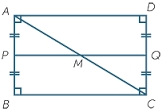# Circumference 8137

One side of the rectangle is 8 cm long. When we enlarge the dimensions of this rectangle four times, we get a rectangle with a circumference of exactly 1m. How many mm does the other side of the unmagnified rectangle measure?

b =  45 mm

### Step-by-step explanation:Did you find an error or inaccuracy? Feel free to write us. Thank you!

Tips for related online calculators
Do you have a linear equation or system of equations and looking for its solution? Or do you have a quadratic equation?

#### Grade of the word problem:

We encourage you to watch this tutorial video on this math problem: